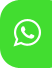## Product Detail## Ignou Solved Assignment For LMT-01/TMA/2019-20

University  IGNOU
Service Type Solved Assignment
Course CTPM
Semester
Short Name or Subject Code Ignou Solved Assignment For LMT-01/TMA/2019-20
Product CTPM of Solved Assignment (IGNOU)
Pattern
Price
Click to view price

1. a) Explain the three main components that form a model of learning. Further, give an example, related to the learning about shape and size, to clarify the nature of each component in the: i) banking model; ii) programming model; iii) constructivist model. (24)

b) Explain what a scheme, and a schema, is. Your explanation should use examples related to the understanding of ‘time’. Further, through an example pertaining to the learning about ‘time’, explain how a scheme can get elaborated through play. (11)

2 a) Outline three constructivist-oriented activities that form a series, to help a child of Class 4 understand the difference between like and unlike fractions. Explain how the activities form a series. (8)

b) Which of the following statements are true? Gives reasons for your answers.

i) Every glide symmetry forms a border.

ii) Only a few children can learn mathematics.

iii) ‘Multiplication of fractions’ is not used in daily life.

iv) Usually, a child has no spatial abilities before she begins school.

v) ‘Data’ is a collection of numbers.

vi) A child-centred classroom is the same as an activity-based classroom. (12)

3. Consider a primary school textbook chapter on decimal fractions. Also look at other chapters on concepts that would be linked with this concept. Is the sequence of linked concepts, as given in the textbook, based on how an adult thinks a child should learn? How different is this sequence from the way it would be if a child-centred curriculum were to be followed? To answer these questions you must interact with several children and teachers. Then explain how you have arrived at your answer to the questions. (10)

4. Explain why it is important to develop the ability to estimate in a child. Further, give a series of three activities, each requiring different levels of abilities of the learner, for developing the skill of estimation. Justify your choice of activities. (15) 4

5. Give an example each in support of the following statements.

i) In some situations the mode of the given data is a better representative value of the data than the mean of this data.

ii) Primary school children need to learn about chance. iii) The product of two integers a and b need not be greater than a or b.

iv) There is more than one way of dividing a three-digit integer by a single digit natural number.

v) Both, inductive and deductive reasoning, are used when applying the principle of mathematical induction. (10)

6. a) What does a teacher lose by not looking carefully at errors made by her learners? Illustrate your explanation with a detailed example related to learning to read maps. (5)

b) Give an example of a good indoor activity to help a child learn to find the area of a 2D object. Explain why it is ‘good’. Further, how would you modify this activity to use it for a class of 30 children?